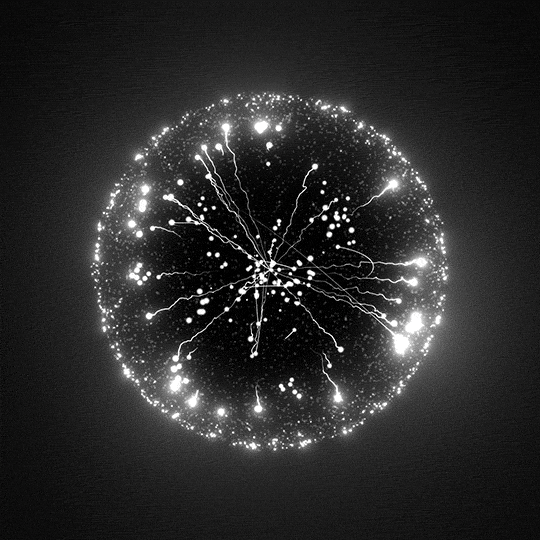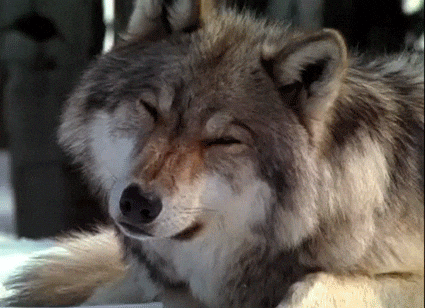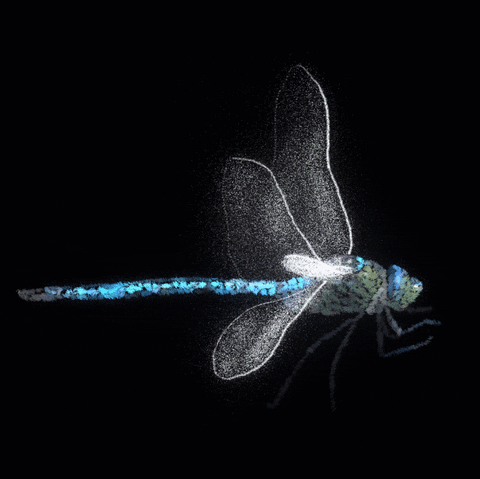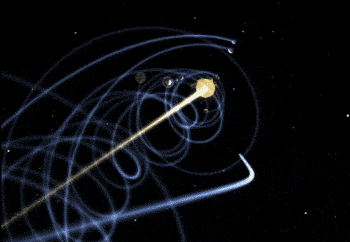# zoofs

Showing:

0

95

3d ago

2

## Package

0

### Categories# zoofs ( Zoo Feature Selection )

`zoofs` is a Python library for performing feature selection using a variety of nature inspired wrapper algorithms. The algorithms range from swarm-intelligence to physics based to Evolutionary. It's an easy to use, flexible and powerful tool to reduce your feature size.

## Documentation

https://jaswinder9051998.github.io/zoofs/

## Whats new in V0.1.2

• now you can pass `timeout` as a parameter to stop operation after the given number of second(s). An amazing alternative to passing number of iterations
• Feature score hashing of visited feature sets to increase the overall performance

## Installation### Using pip

Use the package manager to install zoofs.

``````pip install zoofs
``````

## Available Algorithms

Algorithm NameClass NameDescriptionReferences doi
Particle Swarm AlgorithmParticleSwarmOptimizationUtilizes swarm behaviourhttps://doi.org/10.1007/978-3-319-13563-2_51
Grey Wolf AlgorithmGreyWolfOptimizationUtilizes wolf hunting behaviourhttps://doi.org/10.1016/j.neucom.2015.06.083
Dragon Fly AlgorithmDragonFlyOptimizationUtilizes dragonfly swarm behaviourhttps://doi.org/10.1016/j.knosys.2020.106131
Genetic Algorithm AlgorithmGeneticOptimizationUtilizes genetic mutation behaviourhttps://doi.org/10.1109/ICDAR.2001.953980
Gravitational AlgorithmGravitationalOptimizationUtilizes newtons gravitational behaviourhttps://doi.org/10.1109/ICASSP.2011.5946916

More algos soon, stay tuned !

## Usage

Define your own objective function for optimization !

``````from sklearn.metrics import log_loss
# define your own objective function, make sure the function receives four parameters,
#  fit your model and return the objective value !
def objective_function_topass(model,X_train, y_train, X_valid, y_valid):
model.fit(X_train,y_train)
P=log_loss(y_valid,model.predict_proba(X_valid))
return P

# import an algorithm !
from zoofs import ParticleSwarmOptimization
# create object of algorithm
algo_object=ParticleSwarmOptimization(objective_function_topass,n_iteration=20,
population_size=20,minimize=True)
import lightgbm as lgb
lgb_model = lgb.LGBMClassifier()
# fit the algorithm
algo_object.fit(lgb_model,X_train, y_train, X_valid, y_valid,verbose=True)
algo_object.plot_history()

``````

### Suggestions for Usage

• As available algorithms are wrapper algos, it is better to use ml models that build quicker, e.g lightgbm, catboost.
• Take sufficient amount for 'population_size' , as this will determine the extent of exploration and exploitation of the algo.
• Ensure that your ml model has its hyperparamters optimized before passing it to zoofs algos.

### objective score plot## Algorithms

### Particle Swarm Algorithm#### class zoofs.ParticleSwarmOptimization(objective_function,n_iteration=50,population_size=50,minimize=True,c1=2,c2=2,w=0.9)

Parameters`objective_function` : user made function of the signature 'func(model,X_train,y_train,X_test,y_test)'.
The function must return a value, that needs to be minimized/maximized.
`n_iteration `: int, default=1000
Number of time the algorithm will run
`timeout`: int = None
Stop operation after the given number of second(s). If this argument is set to None, the operation is executed without time limitation and n_iteration is followed
`population_size` : int, default=50
Total size of the population
`minimize `: bool, default=True
Defines if the objective value is to be maximized or minimized
`c1` : float, default=2.0
first acceleration coefficient of particle swarm
`c2` : float, default=2.0
second acceleration coefficient of particle swarm
`w` : float, default=0.9
weight parameter
Attributes`best_feature_list` : array-like
Final best set of features

#### Methods

MethodsClass Name
fitRun the algorithm
plot_historyPlot results achieved across iteration

#### fit(model,X_train, y_train, X_test, y_test,verbose=True)

Parameters`model` :
machine learning model's object
`X_train` : pandas.core.frame.DataFrame of shape (n_samples, n_features)
Training input samples to be used for machine learning model
`y_train` : pandas.core.frame.DataFrame or pandas.core.series.Series of shape (n_samples)
The target values (class labels in classification, real numbers in regression).
`X_valid` : pandas.core.frame.DataFrame of shape (n_samples, n_features)
Validation input samples
`y_valid` : pandas.core.frame.DataFrame or pandas.core.series.Series of shape (n_samples)
The Validation target values .
`verbose` : bool,default=True
Print results for iterations
Returns`best_feature_list ` : array-like
Final best set of features

#### plot_history()

Plot results across iterations

#### Example

``````from sklearn.metrics import log_loss
# define your own objective function, make sure the function receives four parameters,
#  fit your model and return the objective value !
def objective_function_topass(model,X_train, y_train, X_valid, y_valid):
model.fit(X_train,y_train)
P=log_loss(y_valid,model.predict_proba(X_valid))
return P

# import an algorithm !
from zoofs import ParticleSwarmOptimization
# create object of algorithm
algo_object=ParticleSwarmOptimization(objective_function_topass,n_iteration=20,
population_size=20,minimize=True,c1=2,c2=2,w=0.9)
import lightgbm as lgb
lgb_model = lgb.LGBMClassifier()
# fit the algorithm
algo_object.fit(lgb_model,X_train, y_train, X_valid, y_valid,verbose=True)
algo_object.plot_history()
``````

### Grey Wolf Algorithm#### class zoofs.GreyWolfOptimization(objective_function,n_iteration=50,population_size=50,minimize=True)

Parameters`objective_function` : user made function of the signature 'func(model,X_train,y_train,X_test,y_test)'.
The function must return a value, that needs to be minimized/maximized.
`n_iteration `: int, default=50
Number of time the algorithm will run
`timeout`: int = None
Stop operation after the given number of second(s). If this argument is set to None, the operation is executed without time limitation and n_iteration is followed
`population_size` : int, default=50
Total size of the population
`minimize `: bool, default=True
Defines if the objective value is to be maximized or minimized
Attributes`best_feature_list` : array-like
Final best set of features

#### Methods

MethodsClass Name
fitRun the algorithm
plot_historyPlot results achieved across iteration

#### fit(model,X_train,y_train,X_valid,y_valid,method=1,verbose=True)

Parameters`model` :
machine learning model's object
`X_train` : pandas.core.frame.DataFrame of shape (n_samples, n_features)
Training input samples to be used for machine learning model
`y_train` : pandas.core.frame.DataFrame or pandas.core.series.Series of shape (n_samples)
The target values (class labels in classification, real numbers in regression).
`X_valid` : pandas.core.frame.DataFrame of shape (n_samples, n_features)
Validation input samples
`y_valid` : pandas.core.frame.DataFrame or pandas.core.series.Series of shape (n_samples)
The Validation target values .
`method` : {1, 2}, default=1
Choose the between the two methods of grey wolf optimization
`verbose` : bool,default=True
Print results for iterations
Returns`best_feature_list ` : array-like
Final best set of features

#### plot_history()

Plot results across iterations

#### Example

``````from sklearn.metrics import log_loss
# define your own objective function, make sure the function receives four parameters,
#  fit your model and return the objective value !
def objective_function_topass(model,X_train, y_train, X_valid, y_valid):
model.fit(X_train,y_train)
P=log_loss(y_valid,model.predict_proba(X_valid))
return P

# import an algorithm !
from zoofs import GreyWolfOptimization
# create object of algorithm
algo_object=GreyWolfOptimization(objective_function_topass,n_iteration=20,
population_size=20,minimize=True)
import lightgbm as lgb
lgb_model = lgb.LGBMClassifier()
# fit the algorithm
algo_object.fit(lgb_model,X_train, y_train, X_valid, y_valid,method=1,verbose=True)
algo_object.plot_history()
``````

### Dragon Fly Algorithm#### class zoofs.DragonFlyOptimization(objective_function,n_iteration=50,population_size=50,minimize=True)

Parameters`objective_function` : user made function of the signature 'func(model,X_train,y_train,X_test,y_test)'.
The function must return a value, that needs to be minimized/maximized.
`n_iteration `: int, default=50
Number of time the algorithm will run
`timeout`: int = None
Stop operation after the given number of second(s). If this argument is set to None, the operation is executed without time limitation and n_iteration is followed
`population_size` : int, default=50
Total size of the population
`minimize `: bool, default=True
Defines if the objective value is to be maximized or minimized
Attributes`best_feature_list` : array-like
Final best set of features

#### Methods

MethodsClass Name
fitRun the algorithm
plot_historyPlot results achieved across iteration

#### fit(model,X_train,y_train,X_valid,y_valid,method='sinusoidal',verbose=True)

Parameters`model` :
machine learning model's object
`X_train` : pandas.core.frame.DataFrame of shape (n_samples, n_features)
Training input samples to be used for machine learning model
`y_train` : pandas.core.frame.DataFrame or pandas.core.series.Series of shape (n_samples)
The target values (class labels in classification, real numbers in regression).
`X_valid` : pandas.core.frame.DataFrame of shape (n_samples, n_features)
Validation input samples
`y_valid` : pandas.core.frame.DataFrame or pandas.core.series.Series of shape (n_samples)
The Validation target values .
`method` : {'linear','random','quadraic','sinusoidal'}, default='sinusoidal'
Choose the between the three methods of Dragon Fly optimization
`verbose` : bool,default=True
Print results for iterations
Returns`best_feature_list ` : array-like
Final best set of features

#### plot_history()

Plot results across iterations

#### Example

``````from sklearn.metrics import log_loss
# define your own objective function, make sure the function receives four parameters,
#  fit your model and return the objective value !
def objective_function_topass(model,X_train, y_train, X_valid, y_valid):
model.fit(X_train,y_train)
P=log_loss(y_valid,model.predict_proba(X_valid))
return P

# import an algorithm !
from zoofs import DragonFlyOptimization
# create object of algorithm
algo_object=DragonFlyOptimization(objective_function_topass,n_iteration=20,
population_size=20,minimize=True)
import lightgbm as lgb
lgb_model = lgb.LGBMClassifier()
# fit the algorithm
algo_object.fit(lgb_model,X_train, y_train, X_valid, y_valid, method='sinusoidal', verbose=True)
algo_object.plot_history()
``````

### Genetic Algorithm#### class zoofs.GeneticOptimization(objective_function,n_iteration=20,population_size=20,selective_pressure=2,elitism=2,mutation_rate=0.05,minimize=True)

Parameters`objective_function` : user made function of the signature 'func(model,X_train,y_train,X_test,y_test)'.
The function must return a value, that needs to be minimized/maximized.
`n_iteration`: int, default=50
Number of time the algorithm will run
`timeout`: int = None
Stop operation after the given number of second(s). If this argument is set to None, the operation is executed without time limitation and n_iteration is followed
`population_size` : int, default=50
Total size of the population
`selective_pressure`: int, default=2
measure of reproductive opportunities for each organism in the population
`elitism`: int, default=2
number of top individuals to be considered as elites
`mutation_rate`: float, default=0.05
rate of mutation in the population's gene
`minimize`: bool, default=True
Defines if the objective value is to be maximized or minimized
Attributes`best_feature_list` : array-like
Final best set of features

#### Methods

MethodsClass Name
fitRun the algorithm
plot_historyPlot results achieved across iteration

#### fit(model,X_train,y_train,X_valid,y_valid,verbose=True)

Parameters`model` :
machine learning model's object
`X_train` : pandas.core.frame.DataFrame of shape (n_samples, n_features)
Training input samples to be used for machine learning model
`y_train` : pandas.core.frame.DataFrame or pandas.core.series.Series of shape (n_samples)
The target values (class labels in classification, real numbers in regression).
`X_valid` : pandas.core.frame.DataFrame of shape (n_samples, n_features)
Validation input samples
`y_valid` : pandas.core.frame.DataFrame or pandas.core.series.Series of shape (n_samples)
The Validation target values .
`verbose` : bool,default=True
Print results for iterations
Returns`best_feature_list ` : array-like
Final best set of features

#### plot_history()

Plot results across iterations

#### Example

``````from sklearn.metrics import log_loss
# define your own objective function, make sure the function receives four parameters,
#  fit your model and return the objective value !
def objective_function_topass(model,X_train, y_train, X_valid, y_valid):
model.fit(X_train,y_train)
P=log_loss(y_valid,model.predict_proba(X_valid))
return P

# import an algorithm !
from zoofs import GeneticOptimization
# create object of algorithm
algo_object=GeneticOptimization(objective_function_topass,n_iteration=20,
population_size=20,selective_pressure=2,elitism=2,
mutation_rate=0.05,minimize=True)
import lightgbm as lgb
lgb_model = lgb.LGBMClassifier()
# fit the algorithm
algo_object.fit(lgb_model,X_train, y_train,X_valid, y_valid, verbose=True)
algo_object.plot_history()
``````

### Gravitational Algorithm#### class zoofs.GravitationalOptimization(self,objective_function,n_iteration=50,population_size=50,g0=100,eps=0.5,minimize=True)

Parameters`objective_function` : user made function of the signature 'func(model,X_train,y_train,X_test,y_test)'.
The function must return a value, that needs to be minimized/maximized.
`n_iteration`: int, default=50
Number of time the algorithm will run
`timeout`: int = None
Stop operation after the given number of second(s). If this argument is set to None, the operation is executed without time limitation and n_iteration is followed
`population_size` : int, default=50
Total size of the population
`g0`: float, default=100
gravitational strength constant
`eps`: float, default=0.5
distance constant
`minimize`: bool, default=True
Defines if the objective value is to be maximized or minimized
Attributes`best_feature_list` : array-like
Final best set of features

#### Methods

MethodsClass Name
fitRun the algorithm
plot_historyPlot results achieved across iteration

#### fit(model,X_train,y_train,X_valid,y_valid,verbose=True)

Parameters`model` :
machine learning model's object
`X_train` : pandas.core.frame.DataFrame of shape (n_samples, n_features)
Training input samples to be used for machine learning model
`y_train` : pandas.core.frame.DataFrame or pandas.core.series.Series of shape (n_samples)
The target values (class labels in classification, real numbers in regression).
`X_valid` : pandas.core.frame.DataFrame of shape (n_samples, n_features)
Validation input samples
`y_valid` : pandas.core.frame.DataFrame or pandas.core.series.Series of shape (n_samples)
The Validation target values .
`verbose` : bool,default=True
Print results for iterations
Returns`best_feature_list ` : array-like
Final best set of features

#### plot_history()

Plot results across iterations

#### Example

``````from sklearn.metrics import log_loss
# define your own objective function, make sure the function receives four parameters,
#  fit your model and return the objective value !
def objective_function_topass(model,X_train, y_train, X_valid, y_valid):
model.fit(X_train,y_train)
P=log_loss(y_valid,model.predict_proba(X_valid))
return P

# import an algorithm !
from zoofs import GravitationalOptimization
# create object of algorithm
algo_object=GravitationalOptimization(objective_function_topass,n_iteration=50,
population_size=50,g0=100,eps=0.5,minimize=True)
import lightgbm as lgb
lgb_model = lgb.LGBMClassifier()
# fit the algorithm
algo_object.fit(lgb_model,X_train, y_train, X_valid, y_valid, verbose=True)
algo_object.plot_history()
``````

## Support `zoofs`

The development of `zoofs` relies completely on contributions.

#### Contributing

Pull requests are welcome. For major changes, please open an issue first to discuss what you would like to change.

Please make sure to update tests as appropriate.

18,08,2021

apache-2.0

## Rate & Review

Great Documentation0
Easy to Use0
Performant0
Highly Customizable0
Bleeding Edge0
Responsive Maintainers0
Poor Documentation0
Hard to Use0
Slow0
Buggy0
Abandoned0
Unwelcoming Community0
100